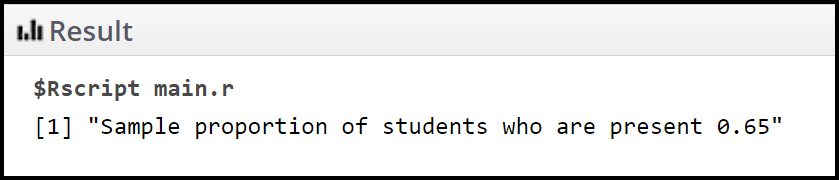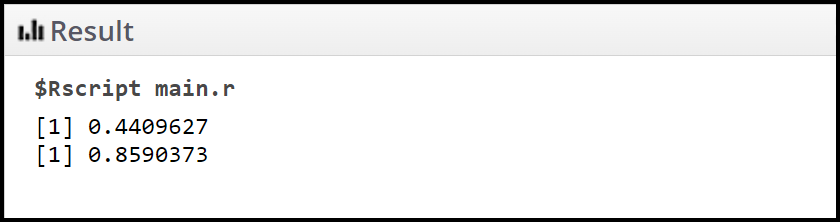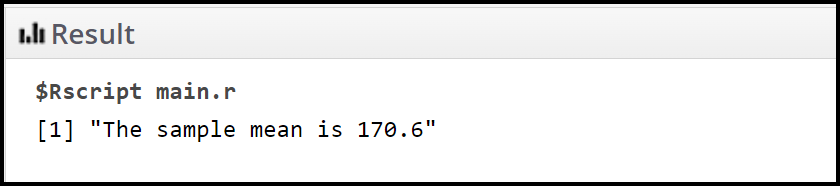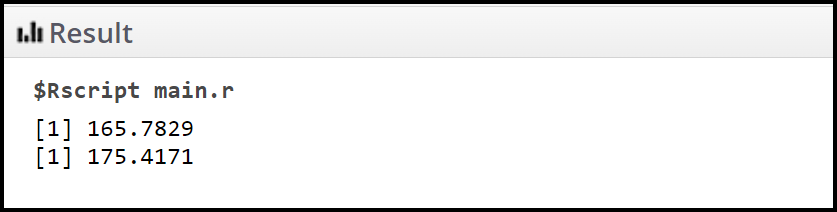Open in App
Not now

# How to Calculate Point Estimates in R?

• Last Updated : 26 Jan, 2022

Point estimation is a technique used to find the estimate or approximate value of population parameters from a given data sample of the population. The point estimate is calculated for the following two measuring parameters:

This article focuses upon how we can calculate point estimates in R Programming Language.

## The point estimate of the population proportion

Point estimation of population proportion can be calculated by using the below mathematical formula,

Syntax: p′ = x / n

Here,

• x : Signifies the number of successes
• n : Signifies the sample size.
• p′ is the point estimate of population proportion

Example:

Let’s say we want to estimate the proportion of students in a class who are present on a particular day. The sample data consist of 20 data elements.

## R

 `# define data``data <- ``c``(``'Present'``, ``'Absent'``, ``'Absent'``, ``'Absent'``,``          ``'Absent'``, ``'Absent'``, ``'Present'``, ``'Present'``, ``          ``'Absent'``, ``'Present'``,``          ``'Present'``, ``'Present'``, ``'Present'``, ``'Present'``, ``          ``'Present'``, ``'Present'``, ``'Absent'``, ``'Present'``, ``          ``'Present'``, ``'Present'``)`` ` `# find total sample size``n <- ``length``(data)`` ` `# find number who are present``k <- ``sum``(data == ``'Present'``) `` ` `# find sample proportion``p <- k/n`` ` `# print``print``(``paste``(``"Sample proportion of students who are present"``, p))`

Output:Example:

Note that we can calculate the 95% confidence interval for the population proportion by using the following source code,

## R

 `# define data``data <- ``c``(``'Present'``, ``'Absent'``, ``'Absent'``, ``'Absent'``,``          ``'Absent'``, ``'Absent'``, ``'Present'``, ``'Present'``, ``          ``'Absent'``, ``'Present'``,``          ``'Present'``, ``'Present'``, ``'Present'``, ``'Present'``,``          ``'Present'``, ``'Present'``, ``'Absent'``, ``'Present'``,``          ``'Present'``, ``'Present'``)`` ` `# find total sample size``total <- ``length``(data)`` ` `# find number who responded 'Yes'``favourable <- ``sum``(data == ``'Present'``) `` ` `# find sample proportion``ans <- favourable/total`` ` `# calculate margin of error``margin <- ``qnorm``(0.975)*``sqrt``(ans*(1-ans)/total)`` ` `# calculate lower and upper bounds of ``# confidence interval``low <- ans - margin``print``(low)`` ` `high <- ans + margin``print``(high)`

Output:Hence, The 95% confidence interval for the population proportion is [0.440, 0.859].

## The point estimate of a population mean

Point estimation of population mean can be calculated by using mean() function in R. The syntax is given below,

Syntax: mean(x, trim = 0, na.rm = FALSE, …)

Here,

• x: It is the input vector
• trim: It is used to drop some observations from both end of the sorted vector
• na.rm: It is used to remove the missing values from the input vector

Example:

Let’s say we want to estimate the population mean of heights of the students in a class. The sample data consist of 20 data elements.

## R

 `#define data``data <- ``c``(170, 180, 165, 170, 165, ``          ``175, 160, 162, 156, 159, ``          ``160, 167, 168, 174, 180, ``          ``167, 169, 180, 190, 195)`` ` `#calculate sample mean``ans <- ``mean``(data, na.rm = ``TRUE``)`` ` `#print the mean height``print``(``paste``(``"The sample mean is"``, ans))`

Output:Hence, The sample means the height is 170.6 cm.

Example:

Note that we can calculate the 95% confidence interval for the population mean by using the following source code,

## R

 `# define data``data <- ``c``(170, 180, 165, 170, 165, 175, ``          ``160, 162, 156, 159, 160, 167,``          ``168, 174, 180, 167, 169, 180,``          ``190, 195)`` ` `# Total number of students``total <- ``length``(data)`` ` `# Point estimate of mean``favourable <- ``mean``(data, na.rm = ``TRUE``)``s <- ``sd``(data)`` ` `# calculate margin of error``margin <- ``qt``(0.975,df=total-1)*s/``sqrt``(total)`` ` `# calculate lower and upper bounds of ``# confidence interval``low <- favourable - margin``print``(low)`` ` `high <- favourable + margin``print``(high)`

Output:Hence, The 95% confidence interval for the population mean is [165.782, 175.417].

My Personal Notes arrow_drop_up